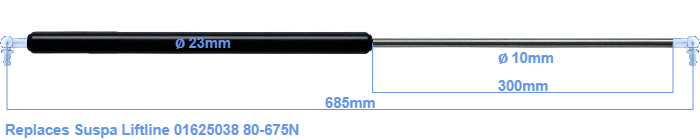# Replacement for Suspa Liftline 01625038 80-675N

\$41.91

Replacement gas spring for the Suspa Liftline 01625038 80-675 Newton. This fits a (possibly already present) ball with a diameter of 10mm. A corresponding ball is included. Brand: Stabilus Industry Line.

Please note:
The diameter of the tube of this replacement gas spring is 23mm instead of 22mm.
 Force Choose an option80 Newton100 Newton120 Newton140 Newton150 Newton160 Newton180 Newton200 Newton220 Newton240 Newton250 Newton260 Newton280 Newton300 Newton320 Newton340 Newton350 Newton360 Newton380 Newton400 Newton420 Newton440 Newton450 Newton460 Newton480 Newton500 Newton520 Newton540 Newton550 Newton560 Newton580 Newton600 Newton620 Newton640 Newton650 Newton660 Newton675 NewtonClear
This gas spring is also known as 16-4 016 25038, 16-4-348-300-A207-B207.The diameter of the black cylinder of this gas spring is 23 millimeter. The diameter of the rod totals 10 mm. The stroke is 300 millimeter long. The total length of this gas strut is 685 mm. This is the distance between the centers of the mounting parts. The length from thread to thread (wihout mounting parts) is 645 mm. The force of this replacement gas spring is 80-675 Newton. Attention: this is not an official Suspa Liftline gas spring, but a replacement gas spring. This a gas spring with the brand Stabilus Industry Line. The gas spring can be used without problems as a replacement, because the dimensions and force almost entirely equal.
Category: